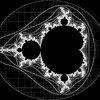## Lissajous XXVI• Available
Products
57
• Artist
Notes

## Artist's Description

// Created with Processing
// Draws a Lissajous figures
// 4 April 2011
// revised 12 April 2018
// Examples

int screen_width = 7700;
int screen_height = 7700;

/* Scale image and reduce by 300 pixels to remove from edge of screen */
int AX = (screen_width / 2) – 600;
int BX = (screen_height / 2) – 600;

float a = 7;
float b = 1;
float x1, y1, x2, y2;

float counter = 0;
int saveCounter = 0;
String file_name;
//
void setup(){
smooth();
fill(255);
strokeWeight(2);
size(7700, 7700);
background(0);
stroke(255);
}
//
void draw(){
//
x1 = (sin(a * counter) * AX) + screen_width / 2;
//
y1 = (sin(b * counter) * BX) + screen_height / 2;
//
counter = counter + 0.5;
//
y2 = screen_width / 2;
//
x2 = screen_height / 2;
//
line(x1,y1,x2,y2);
ellipse((sin(a * counter) * AX) + screen_width / 2 , (sin(b * counter) * BX) + screen_height / 2, 50, 50);
//

println(counter); // saves at 595.0

saveCounter = saveCounter + 1;
if (saveCounter == 1190) { // was 720
String file_name = “Lissajou_a=” + str(a) + “_b=” + str(b) + “-9_7700.png”;
save(file_name);
noLoop();
exit();
}
}

void mousePressed() {
save(“test-9-” + saveCounter + “.png”);
// exit();
}